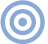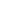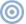# Reference

## Euphonic Distortion: Naughty but Nice? Crunching the Numbers

Sidebar: Crunching the Numbers

To add a desired pattern of nonlinear distortion to a signal, you must first calculate the required polynomial expression relating output amplitude (y) to input amplitude (x), which takes the general form

y = ax + bx2 + cx3 + dx4 + ...

where a, b, c, d, etc., are parameters that determine the pattern of nonlinearity. This equation, called the transfer characteristic, is often plotted as a graph with input voltage along the horizontal axis and output voltage along the vertical axis.

The values of the parameters can be calculated from the harmonic amplitudes in a conventional sinewave distortion measurement by using standard trigonometrical identities, of which the simplest is

cos2θ = 2(cosθ)2 – 1

From this it follows that to add 10% (0.1 x cos2θ) second harmonic distortion to the input signal, the transfer characteristic must be

y = x + 0.2x2 – 0.1

and the parameters are therefore a = 1, b = 0.2. Note that in this case, as with all even-order nonlinearities, a DC offset is also added to the signal, here with the value –0.1.

Similar identities can be written for the other harmonics, although the equations become more complex the higher in order you go. The identity for the fifth harmonic, for instance, is

cos5θ = 5cosθ20(cosθ)3 + 16(cosθ)5

and the transfer characteristic for adding 10% fifth harmonic distortion is therefore

y = 1.5x2x3 + 1.6x5

Note two important features of this relationship. First, the fifth-order nonlinearity modifies the level of the input signal within the output, in this instance increasing it by 50% (+3.5dB). This is a feature of all odd-order distortions (third, fifth, seventh, ninth, etc.). Second, the transfer characteristic includes a cubic factor (–2x3), which on its own would give rise to third-order distortion. This has an important ramification for signals of varying amplitude, such as music, making it impossible to generate fifth-order harmonic and intermodulation products in isolation. In fact, this is true for all nonlinearities of fourth order or higher. Only second- and third-order nonlinearities can be generated independently. This is not a quirk of the math: it reflects the behavior of real circuits.

This process of determining the required trig identities can be continued to whatever order of nonlinearity is desired. I stopped at the 20th. Combining terms from all the identities then allows any desired pattern of nonlinearity to be imposed on a signal. (Hint: If you're going to do this yourself, obtain or get access to Mathcad. I calculated the identities up to the eighth order by hand—a tedious process—before realizing that Mathcad will do it for you at a mouse click.)

I've written a freeware utility, AddDistortion (downloadable from my website), that will add a specified pattern of distortion to a mono 16-bit/44.1kHz WAV file of up to 60 seconds in length. The desired nonlinearity is defined by specifying the relative amplitude of each harmonic, from the second to the 20th, for a full-scale sine input. These amplitudes can be determined from an actual harmonic-distortion measurement, or contrived to fit a desired pattern. Different cosine polarity (plus or minus) can be also specified for each harmonic. Although this information isn't usually available in measurement data, the pattern of harmonic polarities can have a marked effect on both the shape of the transfer characteristic and how the distortion spectrum varies with signal level. There is no space to explore this issue further here, but those interested can read more about it elsewhere (footnote 1).

Once the relative levels and polarities of the harmonics have been specified, AddDistortion upsamples the signal by 24x (in three stages: 2x, 3x, 4x) to prevent distortion products being aliased, calculates the transfer characteristic, and uses this to define the value of the equivalent output sample for each quantization step in the 16-bit input signal, from –32768 to +32767. The results are stored in a lookup table, so that the appropriate output-sample value can be specified immediately on reading each input sample. Once all the output values have been determined, the resulting signal is downsampled by a factor of 24 to return the sampling rate to 44.1kHz, and its gain adjusted, if necessary, to prevent clipping. Triangular probability density function (TPDF) dither is added prior to requantization and the output file is then written. In addition, AddDistortion generates a Windows enhanced metafile containing graphs of the transfer characteristic and autoscaled transfer characteristic error.

An inherent limitation of AddDistortion is that it can only simulate nonlinearities for which exists a one-to-one mapping between input and output. This is equivalent to saying that it cannot model the distortion that results from the mechanical or magnetic hysteresis generally found in loudspeakers and transformers. Hysteresis is beyond the scope of this piece; I hope to return to it on another occasion. Within this limitation, AddDistortion accurately mimics the harmonic and intermodulation distortion added by a given static transfer characteristic. It cannot, as it stands, model frequency-dependent nonlinear behavior or nonlinearity that is time-dependent, both of which would be necessary to create a complete simulation of any real audio component. But it is quite good enough for investigating the subjective consequences of different harmonic patterns.—Keith Howard

Footnote 1: Keith Howard, "Phase Contrast," Hi-Fi News, July 2003.ARTICLE CONTENTSX